•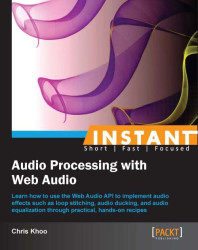#### Instant Audio Processing with Web Audio#### Overview of this book

Web Audio is an upcoming industry standard for web audio processing. Using the API, developers today can develop web games and applications with real-time audio effects to rival their desktop counterparts. Instant Audio Processing with Web Audio is your hands-on guide to the Web Audio API. Using clear, step-by-step exercises, this book explores the API and how to apply it to produce real-time audio effects such as audio stitching, audio ducking, and audio equalization. This book is an in-depth study of the Web Audio API. Through a series of practical, step-by-step exercises, this book will guide you through the basics of playing audio all the way to the task of building a 5-band audio equalizer. Along the way, we'll learn how to utilize Web Audio's scripting functionality to build real-time audio effects such as audio stitching and ducking. Then, we'll use this knowledge to build a basic audio layer step-by-step which can be used in our web applications/games. With its in-depth coverage of the Web Audio API and its practical advice on various audio implementation scenarios, Instant Audio Processing with Web Audio How-to is your ultimate guide to Web Audio.
Instant Audio Processing with Web AudioCreditswww.PacktPub.comPrefaceFree Chapter
Instant Audio Processing with Web AudioOne of the big advantages of using Web Audio is its built-in supporting for constructing sophisticated audio effects in real time. In this recipe, we'll use Web Audio's `BiquadFilterNode` functionality to build a 5-band equalizer. The implementation includes a real-time equalizer frequency response chart display so that we can visualize the equalizer's impact on the sound output.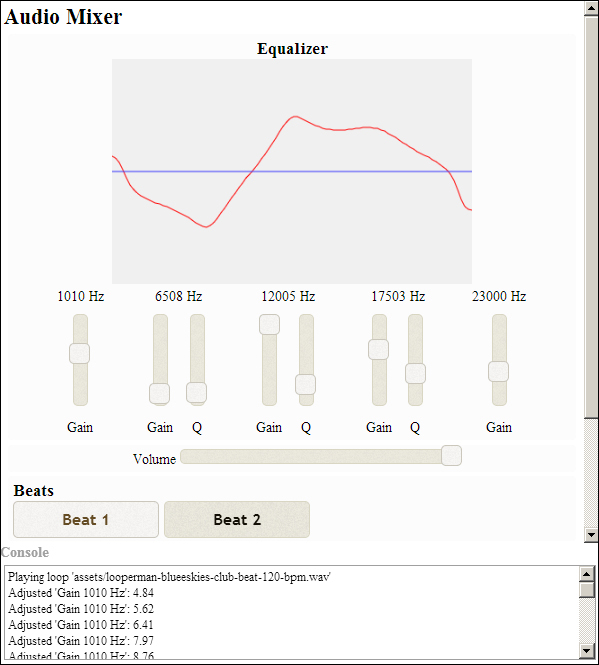This time, we're building our recipe from an audio mixer template so that we can focus specifically on the equalizer implementation (you'll find the template source code in the code bundle at `tools/` `AudioMixerTemplate`). I recommend familiarizing yourself with its implementation—the implementation uses the Web Audio techniques and features we've covered so far.

The complete source code for this recipe is available in the code bundle at `recipes/Recipe6_1`.

### How to do it...

1. Start with a clean copy of the audio mixer template. The template bundle is located at `tools/AudioMixerTemplate` in the code bundle.

2. Open `index.html` in a text editor.

3. We'll add our equalizer controls to the HTML section:

```<div id="appwindow">
<h2>Audio Mixer</h2>
<form>
<div class="audiopanel" style="text-align: center;">
<h3>Equalizer</h3>
<canvas id="eqcanvas" width="400" height="250">
</canvas><br>
<div style="display: inline-block; margin: 0 20px;">
<span id="eq_label_0"></span><br>
<div style="display: inline-block;">
<span id="eq_gain_0" style="display: inline-block; height: 100px; margin: 10px;"></span><br>
<span>Gain</span>
</div>
</div>
<div style="display: inline-block; margin: 0 20px;">
<span id="eq_label_1"></span><br>
<div style="display: inline-block;">
<span id="eq_gain_1" style="display: inline-block; height: 100px; margin: 10px;"></span><br>
<span>Gain</span>
</div>
<div style="display: inline-block;">
<span id="eq_q_1" style="display: inline-block; height: 100px; margin: 10px;"></span><br>
<span>Q</span>
</div>
</div>
<div style="display: inline-block; margin: 0 20px;">
<span id="eq_label_2"></span><br>
<div style="display: inline-block;">
<span id="eq_gain_2" style="display: inline-block; height: 100px; margin: 10px;"></span><br>
<span>Gain</span>
</div>
<div style="display: inline-block;">
<span id="eq_q_2" style="display: inline-block; height: 100px; margin: 10px;"></span><br>
<span>Q</span>
</div>
</div>
<div style="display: inline-block; margin: 0 20px;">
<span id="eq_label_3"></span><br>
<div style="display: inline-block;">
<span id="eq_gain_3" style="display: inline-block; height: 100px; margin: 10px;"></span><br>
<span>Gain</span>
</div>
<div style="display: inline-block;">
<span id="eq_q_3" style="display: inline-block; height: 100px; margin: 10px;"></span><br>
<span>Q</span>
</div>
</div>
<div style="display: inline-block; margin: 0 20px;">
<span id="eq_label_4"></span><br>
<div style="display: inline-block;">
<span id="eq_gain_4" style="display: inline-block; height: 100px; margin: 10px;"></span><br>
<span>Gain</span>
</div>
</div>
</div>

...

</form>
</div>```
4. We'll add the equalizer functionality to `AudioLayer`. In its class constructor, we integrate the equalizer node instances into the audio layer node graph:

```function AudioLayer( audioContext ) {
this.audioContext = audioContext;

// Create the volume GainNode
this.volNode = audioContext.createGain();

// Expose the gain control
this.gain = this.volNode.gain;

// Calculate the frequency metrics
var EQ_FREQ_MARGIN = 1000;  // margin for equalizer range
var NODES_NUM = 5;          // number of equalizer nodes

var freqMin = tempFilter.frequency.minValue
+ EQ_FREQ_MARGIN;
var freqMax = tempFilter.frequency.maxValue
- EQ_FREQ_MARGIN;
var freqStep = (freqMax - freqMin) / (NODES_NUM - 1);

// Create the equalizer nodes to cover the
// frequency spectrum evenly
this.eqNodes = [];
this.eqParms = [];
for( var nodeIndex = 0;
nodeIndex < NODES_NUM;
nodeIndex++ ) {
// Set up the filter

eqNode.frequency.value =
Math.round(freqMin + (nodeIndex * freqStep));

if( nodeIndex == 0 ) {
// Use a low shelf filter for the lowest filter
eqNode.type = "lowshelf";
} else if( nodeIndex == NODES_NUM - 1 ) {
// Use a high shelf filter for the lowest filter
eqNode.type = "highshelf";
} else {
eqNode.type = "peaking";
}

// Connect to the previous node

// Keep track of the node
this.eqNodes.push( eqNode );

// Add the tweakable audio parameters to the
// equalizer parameter array
this.eqParms.push({
frequency:  eqNode.frequency,
Q:          eqNode.Q,
gain:       eqNode.gain
} );

// Keep track of the head node
}

// Connect the volume control to the last head node
}```
5. We'll add the function `AudioLayer.getEqResponse()` to return the equalizer's frequency response graph:

```AudioLayer.prototype.getEqResponse = function( freqs ) {
var magCombined = new Float32Array( freqs.length );

// Get the frequency response from all the eq nodes
var eqNodes = this.eqNodes;
var magCurr = new Float32Array( freqs.length );
var phaseCurr = new Float32Array( freqs.length );
for(var eqIndex=0; eqIndex<eqNodes.length; eqIndex++ ) {
eqNodes[ eqIndex ].getFrequencyResponse(
freqs,
magCurr,
phaseCurr );

// Combine the node magnitudes
for( var freqIndex = 0;
freqIndex < freqs.length;
freqIndex++ ) {
var magDb = Math.log(magCurr[ freqIndex ]) * 20;
magCombined[ freqIndex ] += magDb;
}
}

return magCombined;
};```
6. In `WebAudioApp`, we add the member function `updateEqGraphic()` for rendering the equalizer's response graph:

```WebAudioApp.prototype.updateEqGraphic = function() {
var FREQ_MIN  = 10;           // Hz
var FREQ_MAX  = Math.round(
this.audioContext.sampleRate * 0.5 );

var MAG_MIN = -80;
var MAG_MAX = 80;

// Build the frequency response sampler list
if( this.eqFreqs == null ) {
var FREQS_NUM = 100;
var FREQ_STEP = (FREQ_MAX - FREQ_MIN)
/ (FREQS_NUM - 1);

this.eqFreqs = new Float32Array( FREQS_NUM );
for( var freqIndex = 0;
freqIndex < FREQS_NUM;
freqIndex++ ) {
this.eqFreqs[freqIndex] = Math.round(
FREQ_MIN + (freqIndex * FREQ_STEP) );
}
}

// If we have an update scheduled, don't do anything
if( this.eqUpdateHandle != null )
return;

// Schedule the graphic update
this.eqUpdateHandle = later( 0, function(){
this.eqUpdateHandle = null;

var canvasCtx       = \$("#eqcanvas")
.getContext( "2d" );
var canvasWidth     = canvasCtx.canvas.width;
var canvasHeight    = canvasCtx.canvas.height;

// Calculate the draw steps
var stepX = canvasWidth / (FREQ_MAX - FREQ_MIN);
var stepY = canvasHeight / (MAG_MAX - MAG_MIN );

// Clear the canvas
canvasCtx.fillStyle = "#f0f0f0";
canvasCtx.fillRect( 0, 0, canvasWidth, canvasHeight );

// Draw the frequency response
var eqFreqs = this.eqFreqs;
var eqMag   = this.musicLayer.getEqResponse(eqFreqs);
var firstPt = true;
canvasCtx.beginPath();
for(var index = 0; index < eqFreqs.length; index++ ) {
var x = Math.round(
(eqFreqs[index] - FREQ_MIN) * stepX );
var y = canvasHeight - Math.round(
(eqMag[index] - MAG_MIN) * stepY );

if( firstPt ) {
firstPt = false;
canvasCtx.moveTo( x, y );
} else {
canvasCtx.lineTo( x, y );
}
}

canvasCtx.strokeStyle = "#ff0000";  // red line
canvasCtx.stroke();

// Draw the neutral response line
var neutralY = canvasHeight -
Math.round( (0 - MAG_MIN) * stepY );

canvasCtx.beginPath();
canvasCtx.moveTo( 0, neutralY );
canvasCtx.lineTo( canvasWidth, neutralY );

canvasCtx.strokeStyle = "#3030ff";  // blue line
canvasCtx.stroke();
}, this );
};```
7. Finally, we link the equalizer's HTML control widgets to its corresponding equalizer node and set up the equalizer response graph rendering in `WebAudioApp.start()`:

```WebAudioApp.prototype.start = function() {

..

// Initialize the volume slider
this.initSlider(
"#musicvol",
this.musicLayer.gain,
0.01,
"music volume" );

// Initialize the equalizer sliders
var eqParms = this.musicLayer.eqParms;
for( var nodeIndex = 0;
nodeIndex < eqParms.length;
nodeIndex++ ) {

var parms = eqParms[ nodeIndex ];
var freqValue = String( parms.frequency.value );
this.initSlider(
"#eq_gain_" + nodeIndex,
parms.gain,
0.01,
"Gain " + freqValue + " Hz",
{
orientation: "vertical"
} );
this.initSlider(
"#eq_q_" + nodeIndex,
parms.Q,
0.01,
"Q " + freqValue + " Hz",
{
orientation: "vertical",
min: 0.25,
max: 2.5
} );

// Set up the label
\$("#eq_label_" + nodeIndex).text( freqValue + " Hz" );
}

// Set up equalizer graphics update on slider change
var me = this;
\$( "[id^=eq_]" ).on( "slide", function() {
me.updateEqGraphic();
} );

// Refresh the equalizer graphics
this.updateEqGraphic();
};```

Launch the application test URL in a web browser (`http://localhost/myaudiomixer`) to see the end result. Have fun playing with the mixer!

### How it works...

Web Audio offers a variety of built-in audio processing nodes which can be combined to build sophisticated audio effects. One of these audio processor node types is `BiquadFilterNode`.

`BiquadFilterNode` is actually a collection of common low order filters. These low order filters form the building blocks for tonal control and more advanced filters. The following is its class definition:

1. The `type` attribute indicates the filter type represented by the instance as shown in the following code. Filter types support low-pass, high-pass, low-shelf, high-shelf, and notch filters. We'll discuss this in detail later:

```interface BiquadFilterNode : AudioNode {

var type:String;```
2. The `frequency`, `detune`, `Q`, and `gain` audio parameters affect how the filter processes its input signal. The units for `frequency`, `detune`, and `gain` are Hz, cents (1/100th of a semitone), and dB (decibels—a logarithmic scale for measuring gain), respectively. The units for `Q` vary depending on the type of filter. In fact, the audio parameters are interpreted differently depending on the type of filter:

```    var frequency:AudioParam;       // in Hz
var detune:AudioParam;          // in Cents
var Q:AudioParam;               // Quality factor
var gain:AudioParam             // in dB```

### Note

The filter's applied frequency is the combined result of the `frequency` and `detune` audio parameters.

3. The `getFrequencyResponse()` method returns the frequency response for a list of frequencies as shown in the following code. Applications specify the list of frequencies (in Hz) to sample through the `frequency` parameter. The function then returns the magnitude and phase values associated with the frequencies through the array parameters `magResponse` and `phaseResponse`. Therefore, it is important that the `frequency`, `magResponse`, and `phaseResponse` arrays are of the same size:

```    function getFrequencyResponse(
frequency:Float32Array,        // in
magResponse:Float32Array,      // out
phaseResponse:Float32Array );  // out
};```

The magnitude values are returned as a gain multiplier while the phase values are returned in radians.

### Tip

Make sure that the `getFrequencyResponse()` parameters are the `Float32Array` objects instead of `Array` objects! When the function parameters are not the `Float32Array` objects, the function seems to operate normally, except that the method will exit without filling `magResponse` and `phaseResponse` with the desired information.

The following table summarizes the `BiquadFilterNode` filters and how their audio parameters are interpreted:

Description

`type`

Interpretation

`Frequency`

`gain`

`Q`

Low-pass filter

`lowpass`

The cutoff frequency

Unused

The frequency drop-off steepness at the cutoff frequency

High-pass filter

`highpass`

The cutoff frequency

Unused

The frequency drop-off steepness at the cutoff frequency

Band-pass filter

`bandpass`

Center of the frequency band

Unused

The width of the frequency band

Notch filter

`notch`

Center of the frequency band

Unused

The width of the frequency band

Low-shelf filter

`lowshelf`

The upper limit of the frequencies boosted/attenuated

The boost or attenuation level

Unused

High-shelf filter

`highshelf`

The lower limit of the frequencies boosted/attenuated

The boost or attenuation level

Unused

Peaking filter

`peaking`

Center of the frequency band which is boosted/attenuated

The boost or attenuation level

The width of the frequency band

All-pass filter

`allpass`

Center frequency where the phase transition occurs

Unused

The transition steepness

The following is the graphical representation of the filter types: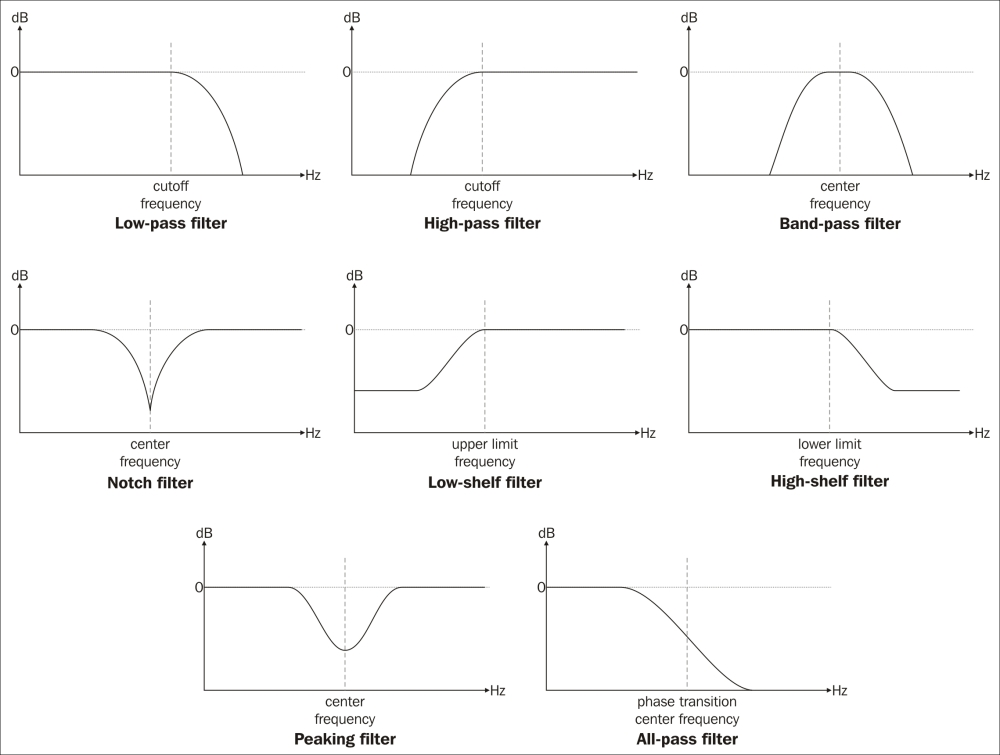In our recipe, we build a 5-band equalizer by:

1. Inserting five `BiquadFilterNode` instances into the node graph—one for each equalizer band. These instances must be inserted in a series so that the output signal is a product of all the `BiquadFilterNode` instances.

2. Exposing each `BiquadFilterNode` instance's `gain` and `Q` audio parameters so that users can tune the equalizer behavior.

The following is the resulting node graph: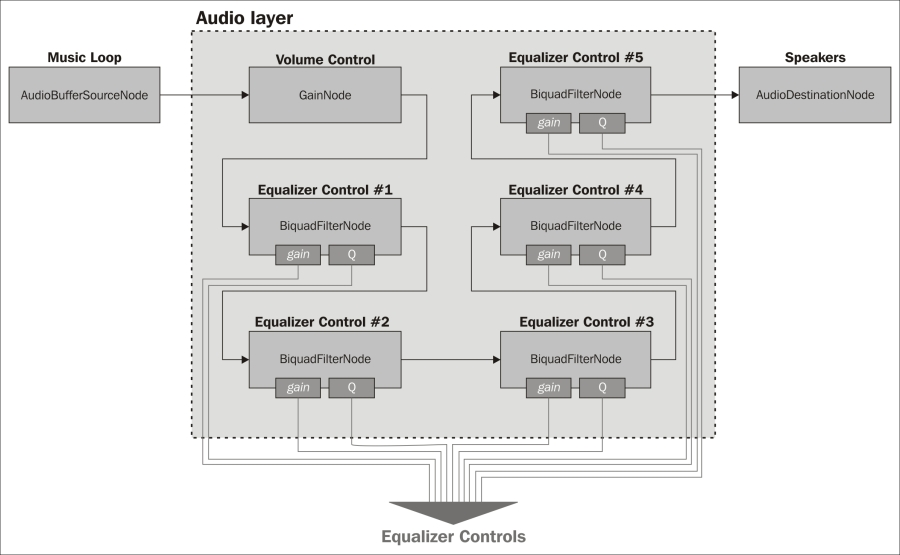Let's take a look at the equalizer set up code in the `AudioLayer` class constructor:

1. First, we'll need to determine the default frequencies for all the equalizer bands. In order to determine this, we'll need to know the supported frequency range. We create a temporary `BiquadFilterNode` instance and sample its `frequency` audio parameter to get the frequency range. This range is reduced by `EQ_FREQ_MARGIN` at both ends yielding the cutoff frequencies for the lowest and highest equalizer bands, `freqMin` and `freqMax`. It's important that the equalizer frequency range has margins on both ends to ensure that the band filters at the ends have enough frequency range to operate properly. Finally, we calculate the default frequency step size between bands and store it in `freqStep`:

```function AudioLayer( audioContext ) {

...
// Calculate the frequency metrics
var EQ_FREQ_MARGIN = 1000;  // margin for equalizer range
var NODES_NUM = 5;          // number of equalizer nodes

var freqMin = tempFilter.frequency.minValue
+ EQ_FREQ_MARGIN;
var freqMax = tempFilter.frequency.maxValue
- EQ_FREQ_MARGIN;
var freqStep = (freqMax - freqMin) / (NODES_NUM - 1);```
2. We instantiate `BiquadFilterNode` for each equalizer band and set its `frequency` audio parameter to its default band frequency:

```    // Create the equalizer nodes to cover the
// frequency spectrum evenly
this.eqNodes = [];
this.eqParms = [];

for( var nodeIndex = 0;
nodeIndex < NODES_NUM;
nodeIndex++ ) {
// Set up the filter
eqNode.frequency.value =
Math.round( freqMin + (nodeIndex * freqStep) );```
3. We use a combination of peaking filters, low-shelf filters, and high-shelf filters to model the equalizer's frequency response as shown in the following code. We use a low-shelf filter and a high-shelf filter for the lowest frequency and the highest frequency equalization bands, respectively. We use peaking filters for all the other bands:

```        if( nodeIndex == 0 ) {
// Use a low shelf filter for the lowest filter
eqNode.type = "lowshelf";
} else if( nodeIndex == NODES_NUM - 1 ) {
// Use a high shelf filter for the lowest filter
eqNode.type = "highshelf";
} else {
eqNode.type = "peaking";
}```

### Tip

Low-shelf/high-shelf versus peaking filters

It's good practice to use low-shelf/high-shelf filters over peaking filters when dealing with filtering at the frequency extremes. In theory, peaking filters should behave like low-shelf/high-shelf filters when used at the frequency extremes. In practice, the peaking filter frequency response deteriorates rapidly as it approaches the extremes. On the other hand, the low-shelf/high-shelf frequency response remains consistent.

4. We connect the `BiquadFilterNode` instances in a series to the audio context's `AudioDestinationNode` instance. Then, we cache each instance's `frequency`, `Q`, and `gain` audio parameters in `AudioLayer.eqParms` so that the applications can access the equalizer controllers directly:

```        // Connect to the previous node

// Keep track of the node
this.eqNodes.push( eqNode );

// Add the tweakable audio parameters to the
// equalizer parameter array
this.eqParms.push({
frequency:  eqNode.frequency,
Q:          eqNode.Q,
gain:       eqNode.gain
} );

// Keep track of the head node
5. Finally, we connect the layer's volume control, `AudioLayer.volNode`, to the `BiquadFilterNode` chain. The volume control is treated as the audio layer's "terminal" `AudioNode` instance—all source `AudioNode` instances must be connected to the volume control node in order to be considered as a part of the `AudioLayer` instance's node graph:

```    }

// Connect the volume control to the last head node
}```

`AudioLayer.getEqResponse()` implements the logic for calculating the equalizer's frequency response:

1. Using `biquadFilterNode.getFreqResponse()`, we retrieve each equalizer band's frequency response for the target frequency list, `freqs`. The method fills `magCurr` with the response magnitude information:

```AudioLayer.prototype.getEqResponse = function( freqs ) {
var magCombined = new Float32Array( freqs.length );

// Get the frequency response from all the eq nodes
var eqNodes = this.eqNodes;
var magCurr = new Float32Array( freqs.length );
var phaseCurr = new Float32Array( freqs.length );
for( var eqIndex = 0;
eqIndex < eqNodes.length;
eqIndex++ ) {

eqNodes[ eqIndex ].getFrequencyResponse(
freqs,
magCurr,
phaseCurr );```
2. We convert each magnitude value from its original gain multiplier value to its dB equivalent:

```        // Combine the node magnitudes
for( var freqIndex = 0;
freqIndex < freqs.length;
freqIndex++ ) {
var magDb = Math.log(magCurr[ freqIndex ]) * 20;```

The formula for converting a gain multiplier to dB is as follows: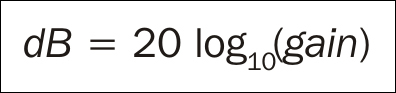3. Then, we sum the magnitude on a per frequency basis. The accumulated magnitude is cached in `magCombined`:

`            magCombined[ freqIndex ] += magDb;`

### Tip

An alternate method for calculating the combined frequency response is to multiply the gain multiplier for each sample frequency together, and then convert the combined gain multiplier into dB.

4. We return `magCombined` once all the `biquadFilterNode` frequency responses have been summed:

```        }
}

return magCombined;
};```

Finally, `WebAudioApp.updateEqGraphic()` renders the equalizer frequency response to an HTML canvas:

1. `FREQ_MIN` and `FREQ_MAX` specify the graph's rendered frequency range as shown in the following code. `FREQ_MAX` is set to the highest supported frequency so that we get a complete frequency response graph. The highest sample frequency that is supported by an `AudioContext` instance is its Nyquist frequency:

```WebAudioApp.prototype.updateEqGraphic = function() {
var FREQ_MIN  = 10;             // Hz
var FREQ_MAX  = Math.round(
this.audioContext.sampleRate * 0.5 );```

### Note

The Nyquist frequency is calculated by dividing the audio context's sample rate in half.

2. The graph renders the frequency response in dB units between the range of `MAG_MIN` and `MAG_MAX`:

```    var MAG_MIN = -80;
var MAG_MAX = 80;```
3. The first time we try to render the response graph, we generate the sample frequency list and store it in `WebAudioApp.eqFreqs` as a `Float32Array` object:

```    // Build the frequency response sampler list
if( this.eqFreqs == null ) {
var FREQS_NUM = 100;
var FREQ_STEP = (FREQ_MAX - FREQ_MIN) / (FREQS_NUM - 1);

this.eqFreqs = new Float32Array( FREQS_NUM );
for( var freqIndex = 0; freqIndex < FREQS_NUM; freqIndex++ )
this.eqFreqs[freqIndex] = Math.round(
FREQ_MIN + (freqIndex * FREQ_STEP) );
}```
4. Because the frequency response retrieval and rendering is a fairly expensive operation, we limit the operation to occur once per update. We enforce this limit by queuing the render operation for the next update and monitoring `WebAudioApp.eqUpdateHandle` to determine when the operation has executed:

```    // If we have an update scheduled, don't do anything
if( this.eqUpdateHandle != null )
return;

// Schedule the graphic update
this.eqUpdateHandle = later( 0, function(){
this.eqUpdateHandle = null;```
5. We start the frequency response graph rendering by clearing the canvas:

```        var canvasCtx = \$("#eqcanvas").getContext( "2d" );
var canvasWidth  = canvasCtx.canvas.width;
var canvasHeight = canvasCtx.canvas.height;

// Calculate the draw steps
var stepX = canvasWidth / (FREQ_MAX - FREQ_MIN);
var stepY = canvasHeight / (MAG_MAX - MAG_MIN );

// Clear the canvas
canvasCtx.fillStyle = "#f0f0f0";
canvasCtx.fillRect( 0, 0, canvasWidth, canvasHeight );```
6. We retrieve the equalizer's frequency response by calling `AudioLayer.getEqResponse()` with the sample frequency list cached in `WebAudioApp.eqFreqs`:

```        // Draw the frequency response
var eqFreqs = this.eqFreqs;
var eqMag = this.musicLayer.getEqResponse( eqFreqs );```
7. We render the response graph as a red line strip:

```        var firstPt = true;
canvasCtx.beginPath();
for(var index = 0; index < eqFreqs.length; index++) {
var x = Math.round(
(eqFreqs[index] - FREQ_MIN) * stepX );
var y = canvasHeight - Math.round(
(eqMag[index] - MAG_MIN) * stepY );
if( firstPt ) {
firstPt = false;
canvasCtx.moveTo( x, y );
} else {
canvasCtx.lineTo( x, y );
}
}

canvasCtx.strokeStyle = "#ff0000";  // red line
canvasCtx.stroke();```
8. Finally, we overlay a blue line indicating the `0` dB position.

```        // Draw the neutral response line
var neutralY = canvasHeight -
Math.round( (0 - MAG_MIN) * stepY );

canvasCtx.beginPath();
canvasCtx.moveTo( 0, neutralY );
canvasCtx.lineTo( canvasWidth, neutralY );

canvasCtx.strokeStyle = "#3030ff";  // blue line
canvasCtx.stroke();
}, this );
};```

### There's more...

Finally we talk about the two most popularly used audio processors in Web Audio.

#### Other built-in Web Audio audio processors

The `biquadFilterNode` and `GainNode` instances are just two of the many built-in audio processing nodes available in Web Audio (volume manipulation is considered a form of audio processing).

The following table highlights some of the more popular audio processor classes:

Class

Description

`PannerNode`

Spatializes an incoming audio signal in 3D space.

`DynamicsCompressorNode`

Compresses the volume range of an incoming audio signal. It accomplishes this by lowering the volume of loud segments and raising the volume of soft segments.

`DelayNode`

Delays the incoming audio signal by a specified time delay.

`ConvolverNode`

Mixes an incoming audio signal with an impulse response waveform. This audio processor is a fundamental building block for reverberation effects.

The following node graph illustrates a reverberation effect constructed using Web Audio's built-in audio processing nodes: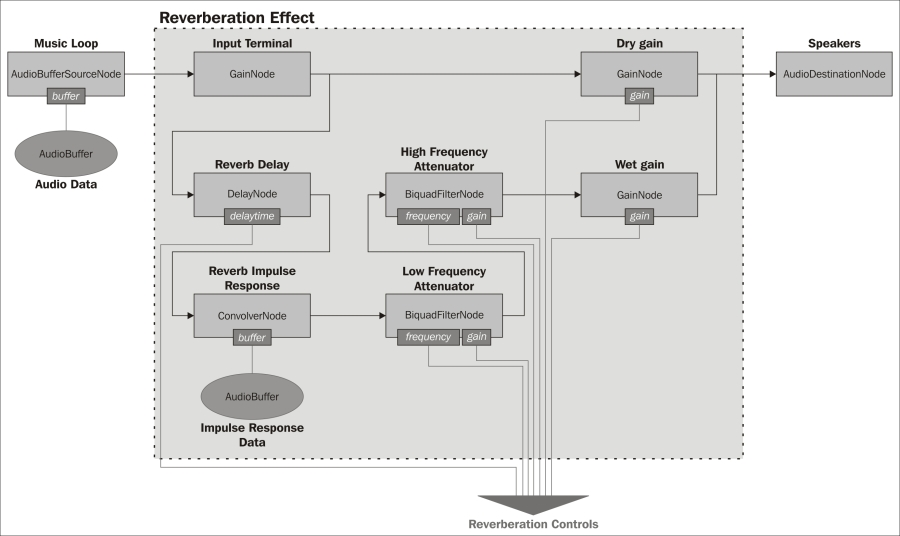The example exposes the most common reverberation control parameters, which are:

• Reverberation delay

• Impulse response

• Reverberation high cut-off frequency

• Reverberation low cut-off frequency

• Dry/wet mix

Unfortunately, a technical implementation of the reverberation audio processor is beyond the scope of this book. Hopefully, the example will serve as an inspiration and jump point to greater and more exciting real-time audio processors on the Web.

This concludes our exploration of the Web Audio API.